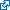### Calculus

Calculus, originally called infinitesimal calculus or "the calculus of infinitesimals", is the mathematical study of continuous change, in the same way that geometry is the study of shape and algebra is the study of generalizations of arithmetic operations. It has two major branches, differential calculus and integral calculus; the former concerns instantaneous rates of change, and the slopes of curves, while integral calculus concerns accumulation of quantities, and areas under or between curves. [Source: Wikipedia]